## de Moivre-Laplace Theorem

The sum of those terms of the Binomial Series offor which the number of successesfalls between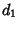and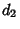is approximately(1)

where(2)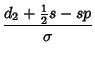(3)(4)

Uspensky (1937) has shown that(5)

where(6)

for.

A Corollary states that the probability thatsuccesses in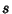trials will differ from the expected valueby more thanis(7)

where(8)

Uspensky (1937) showed that(9)

where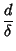(10)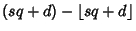(11)(12)

and(13)

for.

References

Uspensky, J. V. Introduction to Mathematical Probability. New York: McGraw-Hill, 1937.